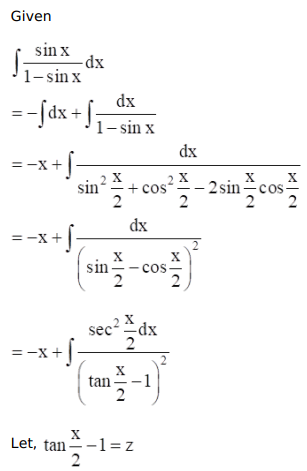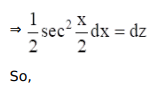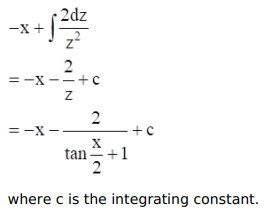# Mark against the correct answer in each of the following:

Question:

Mark $(\sqrt{)}$ against the correct answer in each of the following:

$\int \frac{\sin x}{(1-\sin x)} d x=?$

A. $-x+\sec x-\tan x+C$

B. $x+\cos x-\sin x+C$

C. $-\log |1-\sin x|+C$

D. none of these

Solution: